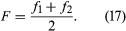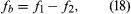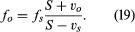## Beats

An important occurrence of the interference of waves is in the phenomenon of beats. In the simplest case, beats result when two sinusoidal sound waves of equal amplitude and very nearly equal frequencies mix. The frequency of the resulting sound (F) would be the average of the two original frequencies (f1 and f2):The amplitude or intensity of the combined signal would rise and fall at a rate (fb) equal to the difference between the two original frequencies,where f1 is greater than f2.

Beats are useful in tuning musical instruments to each other: the farther the instruments are out of tune, the faster the beats. Other types of beats are also of interest. Second-order beats occur between the two notes of a mistuned octave, and binaural beats involve beating between tones presented separately to the two ears, so that they do not mix physically.

## The Doppler effect

The Doppler effect is a change in the frequency of a tone that occurs by virtue of relative motion between the source of sound and the observer. When the source and the observer are moving closer together, the perceived frequency is higher than the normal frequency, or the frequency heard when the observer is at rest with respect to the source. When the source and the observer are moving farther apart, the perceived frequency is lower than the normal frequency. For the case of a moving source, one example is the falling frequency of a train whistle as the train passes a crossing. In the case of a moving observer, a passenger on the train would hear the warning bells at the crossing drop in frequency as the train speeds by.

For the case of motion along a line, where the source moves with speed vs and the observer moves with speed vo through still air in which the speed of sound is S, the general equation describing the change in frequency heard by the observer isIn this equation the speeds of the source and the observer will be negative if the relative motion between the source and observer is moving them apart, and they will be positive if the source and observer are moving together.

From this equation, it can be deduced that a Doppler effect will always be heard as long as the relative speed between the source and observer is less than the speed of sound. The speed of sound is constant with respect to the air in which it is propagating, so that, if the observer moves away from the source at a speed greater than the speed of sound, nothing will be heard. If the source and the observer are moving with the same speed in the same direction, vo and vs will be equal in magnitude but with the opposite sign; the frequency of the sound will therefore remain unchanged, like the sound of a train whistle as heard by a passenger on the moving train.

## Shock waves

If the speed of the source is greater than the speed of sound, another type of wave phenomenon will occur: the sonic boom. A sonic boom is a type of shock wave that occurs when waves generated by a source over a period of time add together coherently, creating an unusually strong sum wave. An analogue to a sonic boom is the V-shaped bow wave created in water by a motorboat when its speed is greater than the speed of the waves. In the case of an aircraft flying faster than the speed of sound (about 1,230 kilometres per hour, or 764 miles per hour), the shock wave takes the form of a cone in three-dimensional space called the Mach cone. The Mach number is defined as the ratio of the speed of the aircraft to the speed of sound. The higher the Mach number—that is, the faster the aircraft—the smaller the angle of the Mach cone.

Sound

## External Websites

Britannica Websites
Articles from Britannica Encyclopedias for elementary and high school students.

## Article Contributors

×
Britannica presents a time-travelling voice experience